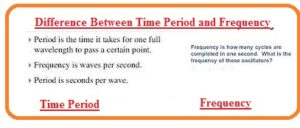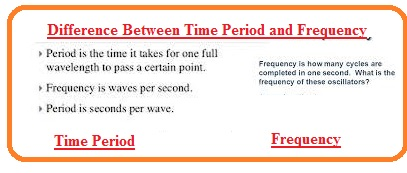Hello guys, I hope you all are doing great. In today’s tutorial, we will have a look at Difference Between Time Period and Frequency. There are 2 most important parameters related to wave first one is a time period and the second one is frequency. These are inverse of one another. The basic difference among them is that the time period is the duration in which the cycle of a wave is completed. While the frequency is a completion of a number of wave cycles in certain time intervals.

As we know that wave is produced due to the vibration of an object. Also, we know that motion ha a relationship with motion. Therefore, any oscillating object consists of some amount of energy and force. So oscillation is known as periodic motion that is repeated after some time interval. In today’s post, we will compare both time periods and frequency and find their differences. So let’s get started with Difference Between Time Period and Frequency.

#### Difference Between Time Period and Frequency

Time Period

• The time interval over which the cycle of the wave is completed is called time period. It is also called the inverse of frequency.
• It is denoted as T.
• It also defines as cycles per second.
• It is the time taken to perform some work
• The time period is larger frequency is less
• The time needed to completer single rotation for the wave is called the period
• Its measuring unit is second.
• It is the inverse of frequency
• Its formula is T=1/f.

Frequency

• The time through which the cycle is repeated is called a period it is the inverse of the frequency
• It is used to discuss the rate of oscillation and vibration in different scientific fields.
• It employed for observation of light frequency in different aspect
• Frequency also called the inverse of the time period.
• One hertz is equal to the complete rotation in one second.
• One complete waveform of the current is called a cycle
• The frequency of ac system is sixty or fifty hertz according to our country
• The range of frequency that can be heard is fifteen hertz to twenty-kilo hertz
• Radiofrequency has thirty to three hundred hertz
• One-half cycle called alternation
• It is denoted as f’.
• F=I/t
• It is measured in hertz.
• It formula is f=v/λ.
• The sound of frequency which we can lies between twenty hertz to twenty-kilo hertz.#### Time Period vs Frequency

Time Period Frequency
Definition: Definition:
It is time required for the completion of one cycle It is a number of repetitive events in unit time
Symbol: Symbol:
T f
Unit: Unit:
Seconds (s) Hertz (Hz)
Formula: Formula:
T = 1/f f = 1/T
Inverse Proportionality: Inverse Proportionality:
These two terms are in inverse relation to each other Time period and frequency are inversely proportional and have an inverse relation
With the increases in time period frequency decreases With frequency increases time period reduces
Relationship Formula: Relationship Formula:
T × f = 1 T × f = 1
Graphical Representation: Graphical Representation:
it is represented by the period of a waveform. it is drawn by complete waveform per unit of time
Examples: Examples:
The time period of a pendulum having ten oscillations in twenty seconds is two seconds. The frequency of a guitar string vibrating 440 times in 1 second is 440 Hz.
Applications: Applications:
Music: They are used to describe the sound pitch Music: Time period and frequency are for tuning the musical devices
Electronics: it is sued in electronic circuits like filters and oscillators Electronics: Used in signal processing and communication systems.
It is used for the study of features of astronomy, like the rotation of different planets and stars. pulsation.  it is used for the detection of and analyzing, like gravitational waves cosmic phenomena, and black holes.

### FAQS

1. Write main difference between time period and frequency.
• The time period is the time necessary for one complete cycle of a repetitive event, whereas frequency is the number of repetitions of a repetitive event per unit of time
1. Write units of time period and frequency.
• frequency unit is Hertz (Hz), and unit of time is seconds (s).
1. Define the formula for calculating time period.
• T = 1/f, where T is the time period and f is the frequency, is the formula for time.
1. What is the formula of frequency?
• f = 1/T, where f represents frequency and T is time period, is the formula for frequency.
1. What is the relationship between time period and frequency?
• The relationship between duration and frequency is negatively correlated. The frequency drops as the time period lengthens and vice versa.
1. How time periods and frequency are used in music?
• When tuning musical instruments, the pitch of a sound is described using time and frequency.
1. How time periods and frequencies used in electronics?
• Electronic circuits like oscillators and filters, as well as signal processing and communication systems, all employ time and frequency.

That is all about differences between time periods and frequency if you have any queries ask in the comments. Thanks for reading. Have a good day.